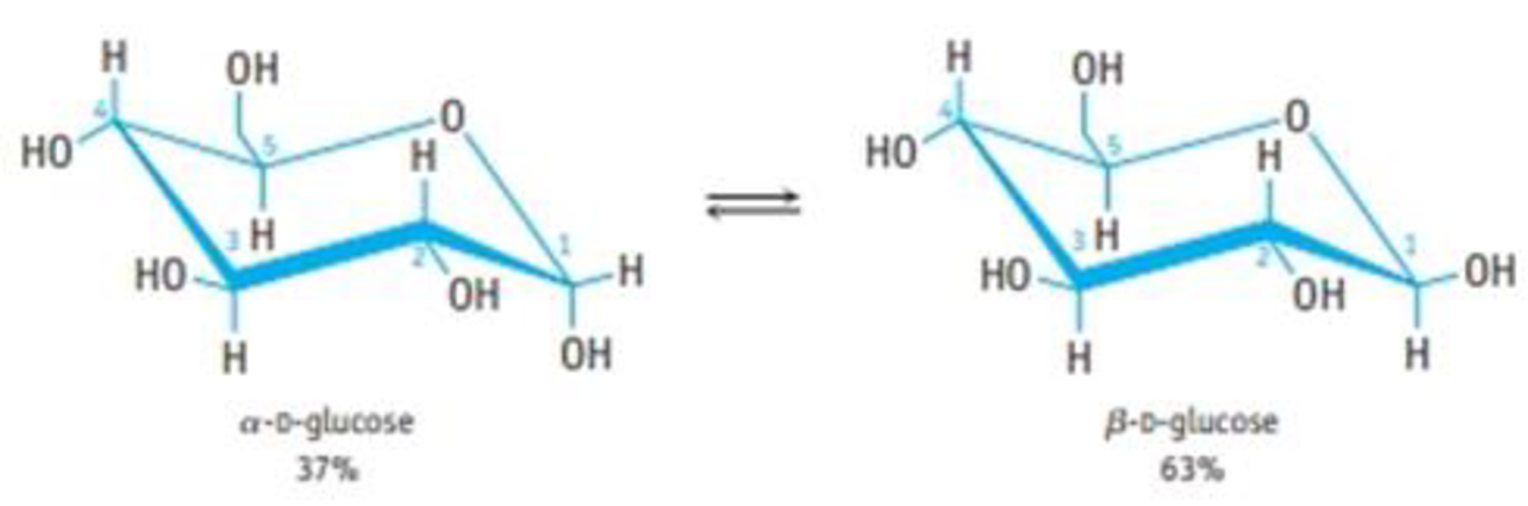Chapter 24, Problem 28GQ

Chapter
Section
Textbook Problem

What is the equilibrium constant for the conversion of α-D-glucose to β-D-glucose in water at room temperature (assume that there is no glucose present in the open-chain structure)?Interpretation Introduction

Interpretation:

The equilibrium constant of conversion of α-D-glucose to β-D-glucose has to be found.

Concept introduction:

Glucose is the simplest carbohydrate. It is a monosaccharide. Glucose exists in two forms that are α-D-glucose and β-D-glucose. The difference between them is the position of hydrogen and hydroxide group on the 1-C atom.

The β-D-glucose is a more stable form of glucose. The conversion of α-D-glucose to β-D-glucose should be spontaneous.

Explanation

The equilibrium constant for the conversion of α-D-glucose to β-D-glucose is calculated below.

Given:

The equilibrium constant for any reaction is equal to the ratio of the concentration of the product over the reaction.

For a general reaction,

AB

The equilibrium constant (K) is given as,

K=[B][A]

The given reaction is,

α-D-glucoseβ-D-glucose

The percentage of α-D-glucose is 37 %.

The percentage of β-D-glucose is 63 %.

The total percentage of both the isomers is 100 %

Still sussing out bartleby?

Check out a sample textbook solution.

See a sample solution

The Solution to Your Study Problems

Bartleby provides explanations to thousands of textbook problems written by our experts, many with advanced degrees!

Get Started